10.3 Polar coordinates  (Page 5/8)

 Page 5 / 8

How are the polar axes different from the x - and y -axes of the Cartesian plane?

Explain how polar coordinates are graphed.

Determine $\text{\hspace{0.17em}}\theta \text{\hspace{0.17em}}$ for the point, then move $\text{\hspace{0.17em}}r\text{\hspace{0.17em}}$ units from the pole to plot the point. If $\text{\hspace{0.17em}}r\text{\hspace{0.17em}}$ is negative, move $\text{\hspace{0.17em}}r\text{\hspace{0.17em}}$ units from the pole in the opposite direction but along the same angle. The point is a distance of $\text{\hspace{0.17em}}r\text{\hspace{0.17em}}$ away from the origin at an angle of $\text{\hspace{0.17em}}\theta \text{\hspace{0.17em}}$ from the polar axis.

How are the points $\text{\hspace{0.17em}}\left(3,\frac{\pi }{2}\right)\text{\hspace{0.17em}}$ and $\text{\hspace{0.17em}}\left(-3,\frac{\pi }{2}\right)\text{\hspace{0.17em}}$ related?

Explain why the points $\text{\hspace{0.17em}}\left(-3,\frac{\pi }{2}\right)\text{\hspace{0.17em}}$ and $\text{\hspace{0.17em}}\left(3,-\frac{\pi }{2}\right)\text{\hspace{0.17em}}$ are the same.

The point $\text{\hspace{0.17em}}\left(-3,\frac{\pi }{2}\right)\text{\hspace{0.17em}}$ has a positive angle but a negative radius and is plotted by moving to an angle of $\text{\hspace{0.17em}}\frac{\pi }{2}\text{\hspace{0.17em}}$ and then moving 3 units in the negative direction. This places the point 3 units down the negative y -axis. The point $\text{\hspace{0.17em}}\left(3,-\frac{\pi }{2}\right)\text{\hspace{0.17em}}$ has a negative angle and a positive radius and is plotted by first moving to an angle of $\text{\hspace{0.17em}}-\frac{\pi }{2}\text{\hspace{0.17em}}$ and then moving 3 units down, which is the positive direction for a negative angle. The point is also 3 units down the negative y -axis.

Algebraic

For the following exercises, convert the given polar coordinates to Cartesian coordinates with $\text{\hspace{0.17em}}r>0\text{\hspace{0.17em}}$ and $\text{\hspace{0.17em}}0\le \theta \le 2\pi .\text{\hspace{0.17em}}$ Remember to consider the quadrant in which the given point is located when determining $\text{\hspace{0.17em}}\theta \text{\hspace{0.17em}}$ for the point.

$\left(7,\frac{7\pi }{6}\right)$

$\left(5,\pi \right)$

$\left(-5,0\right)$

$\left(6,-\frac{\pi }{4}\right)$

$\left(-3,\frac{\pi }{6}\right)$

$\left(-\frac{3\sqrt{3}}{2},-\frac{3}{2}\right)$

$\left(4,\frac{7\pi }{4}\right)$

For the following exercises, convert the given Cartesian coordinates to polar coordinates with $\text{\hspace{0.17em}}r>0,\text{\hspace{0.17em}}\text{\hspace{0.17em}}0\le \theta <2\pi .\text{\hspace{0.17em}}$ Remember to consider the quadrant in which the given point is located.

$\left(4,2\right)$

$\left(-4,6\right)$

$\left(3,-5\right)$

$\left(\sqrt{34},5.253\right)$

$\left(-10,-13\right)$

$\left(8,8\right)$

$\left(8\sqrt{2},\frac{\pi }{4}\right)$

For the following exercises, convert the given Cartesian equation to a polar equation.

$x=3$

$y=4$

$r=4\mathrm{csc}\theta$

$y=4{x}^{2}$

$y=2{x}^{4}$

$r=\sqrt{\frac{sin\theta }{2co{s}^{4}\theta }}$

${x}^{2}+{y}^{2}=4y$

${x}^{2}+{y}^{2}=3x$

$r=3\mathrm{cos}\theta$

${x}^{2}-{y}^{2}=x$

${x}^{2}-{y}^{2}=3y$

$r=\frac{3\mathrm{sin}\theta }{\mathrm{cos}\left(2\theta \right)}$

${x}^{2}+{y}^{2}=9$

${x}^{2}=9y$

$r=\frac{9\mathrm{sin}\theta }{{\mathrm{cos}}^{2}\theta }$

${y}^{2}=9x$

$9xy=1$

$r=\sqrt{\frac{1}{9\mathrm{cos}\theta \mathrm{sin}\theta }}$

For the following exercises, convert the given polar equation to a Cartesian equation. Write in the standard form of a conic if possible, and identify the conic section represented.

$r=3\mathrm{sin}\text{\hspace{0.17em}}\theta$

$r=4\mathrm{cos}\text{\hspace{0.17em}}\theta$

${x}^{2}+{y}^{2}=4x\text{\hspace{0.17em}}$ or $\text{\hspace{0.17em}}\frac{{\left(x-2\right)}^{2}}{4}+\frac{{y}^{2}}{4}=1;$ circle

$r=\frac{4}{\mathrm{sin}\text{\hspace{0.17em}}\theta +7\mathrm{cos}\text{\hspace{0.17em}}\theta }$

$r=\frac{6}{\mathrm{cos}\text{\hspace{0.17em}}\theta +3\mathrm{sin}\text{\hspace{0.17em}}\theta }$

$3y+x=6;\text{\hspace{0.17em}}$ line

$r=2\mathrm{sec}\text{\hspace{0.17em}}\theta$

$r=3\mathrm{csc}\text{\hspace{0.17em}}\theta$

$y=3;\text{\hspace{0.17em}}$ line

$r=\sqrt{r\mathrm{cos}\text{\hspace{0.17em}}\theta +2}$

${r}^{2}=4\mathrm{sec}\text{\hspace{0.17em}}\theta \text{\hspace{0.17em}}\mathrm{csc}\text{\hspace{0.17em}}\theta$

$xy=4;\text{\hspace{0.17em}}$ hyperbola

$r=4$

${r}^{2}=4$

${x}^{2}+{y}^{2}=4;\text{\hspace{0.17em}}$ circle

$r=\frac{1}{4\mathrm{cos}\text{\hspace{0.17em}}\theta -3\mathrm{sin}\text{\hspace{0.17em}}\theta }$

$r=\frac{3}{\mathrm{cos}\text{\hspace{0.17em}}\theta -5\mathrm{sin}\text{\hspace{0.17em}}\theta }$

$x-5y=3;\text{\hspace{0.17em}}$ line

Graphical

For the following exercises, find the polar coordinates of the point.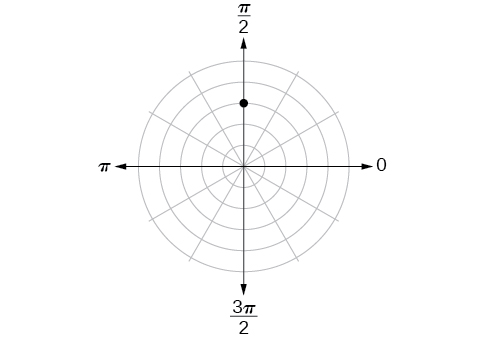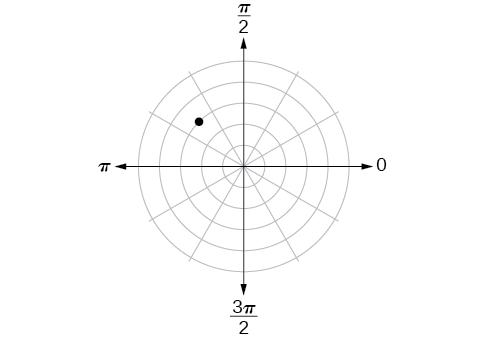$\left(3,\frac{3\pi }{4}\right)$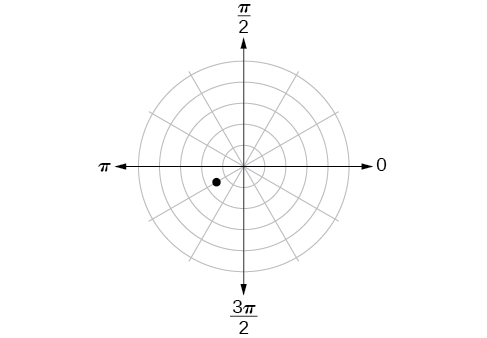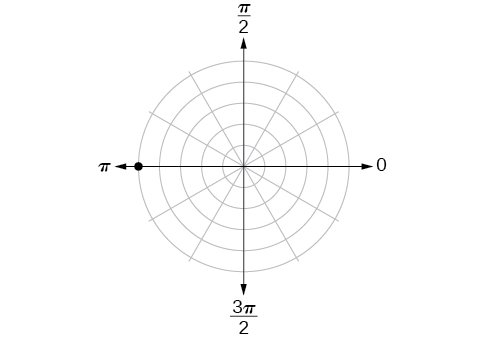$\left(5,\pi \right)$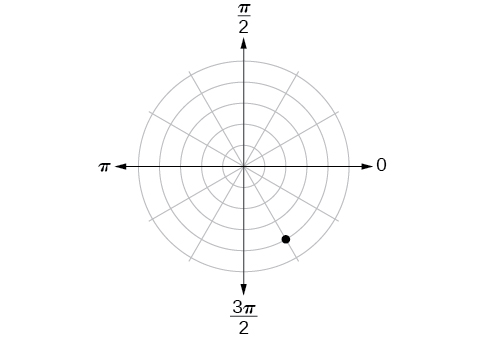For the following exercises, plot the points.

$\left(-2,\frac{\pi }{3}\right)$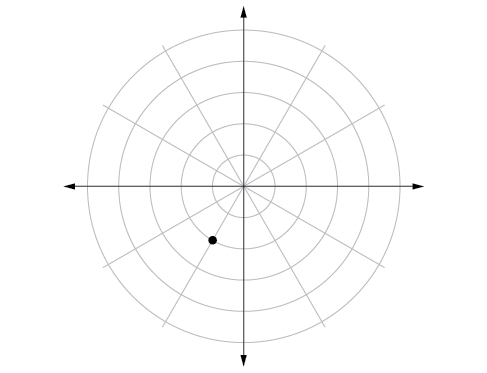$\left(-1,-\frac{\pi }{2}\right)$

$\left(3.5,\frac{7\pi }{4}\right)$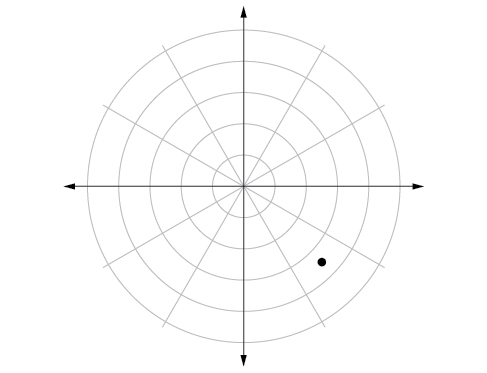$\left(-4,\frac{\pi }{3}\right)$

$\left(5,\frac{\pi }{2}\right)$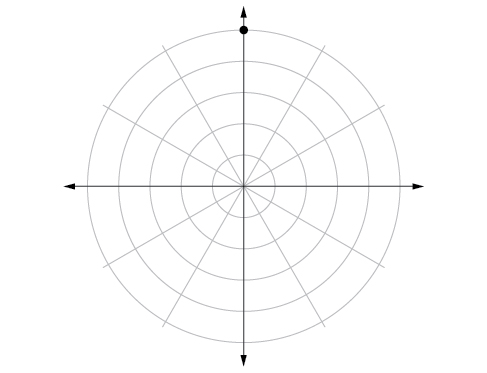$\left(4,\frac{-5\pi }{4}\right)$

$\left(3,\frac{5\pi }{6}\right)$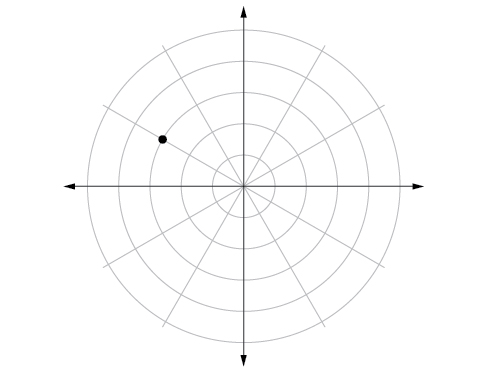$\left(-1.5,\frac{7\pi }{6}\right)$

$\left(-2,\frac{\pi }{4}\right)$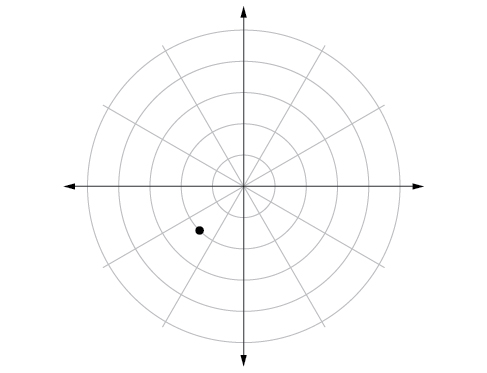$\left(1,\frac{3\pi }{2}\right)$

For the following exercises, convert the equation from rectangular to polar form and graph on the polar axis.

$5x-y=6$

$r=\frac{6}{5\mathrm{cos}\theta -\mathrm{sin}\theta }$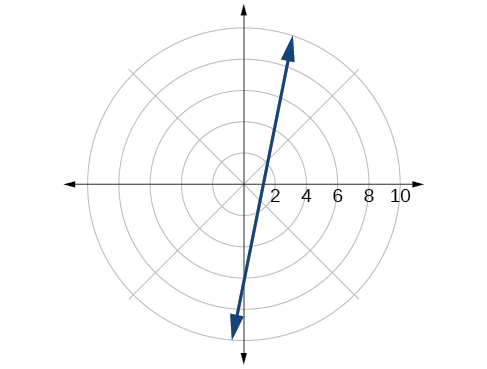$2x+7y=-3$

${x}^{2}+{\left(y-1\right)}^{2}=1$

$r=2\mathrm{sin}\theta$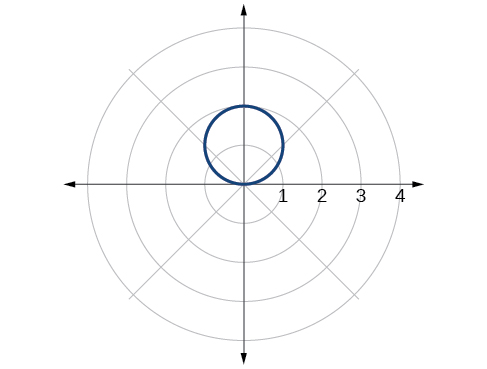${\left(x+2\right)}^{2}+{\left(y+3\right)}^{2}=13$

$x=2$

$r=\frac{2}{\mathrm{cos}\theta }$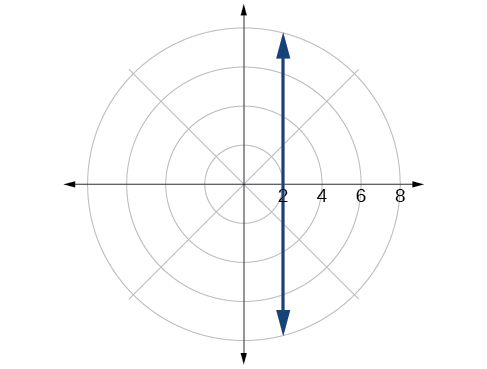${x}^{2}+{y}^{2}=5y$

${x}^{2}+{y}^{2}=3x$

$r=3\mathrm{cos}\theta$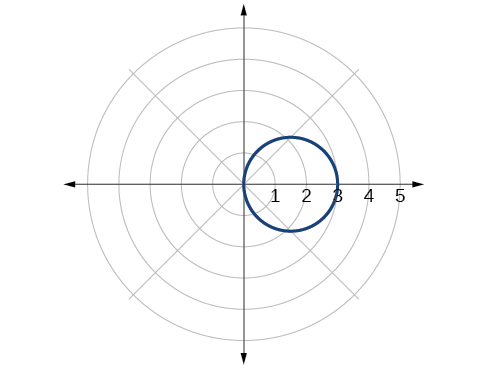For the following exercises, convert the equation from polar to rectangular form and graph on the rectangular plane.

$r=6$

$r=-4$

${x}^{2}+{y}^{2}=16$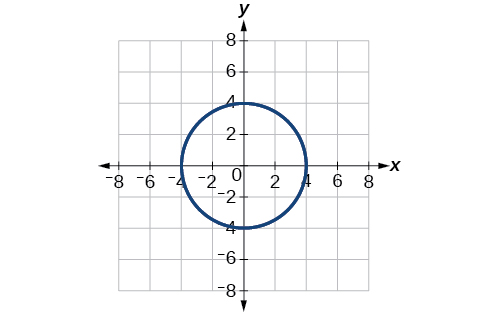$\theta =-\frac{2\pi }{3}$

$\theta =\frac{\pi }{4}$

$y=x$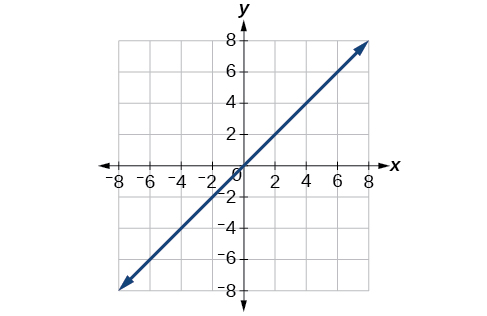$r=\mathrm{sec}\text{\hspace{0.17em}}\theta$

$r=-10\mathrm{sin}\text{\hspace{0.17em}}\theta$

${x}^{2}+{\left(y+5\right)}^{2}=25$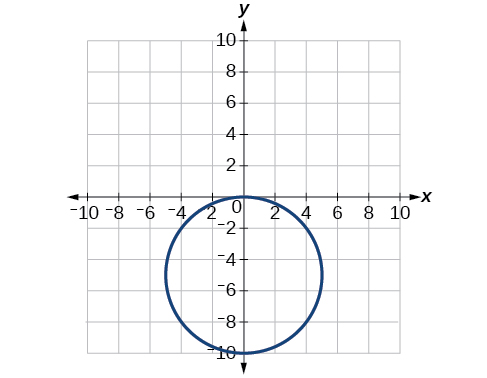$r=3\mathrm{cos}\text{\hspace{0.17em}}\theta$

Technology

Use a graphing calculator to find the rectangular coordinates of $\text{\hspace{0.17em}}\left(2,-\frac{\pi }{5}\right).\text{\hspace{0.17em}}$ Round to the nearest thousandth.

$\left(1.618,-1.176\right)$

Use a graphing calculator to find the rectangular coordinates of $\text{\hspace{0.17em}}\left(-3,\frac{3\pi }{7}\right).\text{\hspace{0.17em}}$ Round to the nearest thousandth.

Use a graphing calculator to find the polar coordinates of $\text{\hspace{0.17em}}\left(-7,8\right)\text{\hspace{0.17em}}$ in degrees. Round to the nearest thousandth.

$\left(10.630,131.186°\right)$

Use a graphing calculator to find the polar coordinates of $\text{\hspace{0.17em}}\left(3,-4\right)\text{\hspace{0.17em}}$ in degrees. Round to the nearest hundredth.

Use a graphing calculator to find the polar coordinates of $\text{\hspace{0.17em}}\left(-2,0\right)\text{\hspace{0.17em}}$ in radians. Round to the nearest hundredth.

$\text{\hspace{0.17em}}\left(2,3.14\right)or\left(2,\pi \right)\text{\hspace{0.17em}}$

Extensions

Describe the graph of $\text{\hspace{0.17em}}r=a\mathrm{sec}\text{\hspace{0.17em}}\theta ;a>0.$

Describe the graph of $\text{\hspace{0.17em}}r=a\mathrm{sec}\text{\hspace{0.17em}}\theta ;a<0.$

A vertical line with $\text{\hspace{0.17em}}a\text{\hspace{0.17em}}$ units left of the y -axis.

Describe the graph of $\text{\hspace{0.17em}}r=a\mathrm{csc}\text{\hspace{0.17em}}\theta ;a>0.$

Describe the graph of $\text{\hspace{0.17em}}r=a\mathrm{csc}\text{\hspace{0.17em}}\theta ;a<0.$

A horizontal line with $\text{\hspace{0.17em}}a\text{\hspace{0.17em}}$ units below the x -axis.

What polar equations will give an oblique line?

For the following exercise, graph the polar inequality.

$r<4$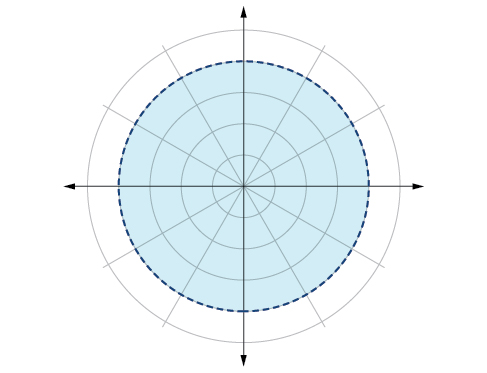$0\le \theta \le \frac{\pi }{4}$

$\theta =\frac{\pi }{4},\text{\hspace{0.17em}}r\text{\hspace{0.17em}}\ge \text{\hspace{0.17em}}2$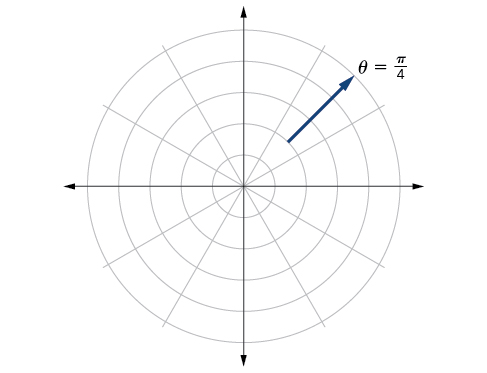$\theta =\frac{\pi }{4},\text{\hspace{0.17em}}r\text{\hspace{0.17em}}\ge -3$

$0\le \theta \le \frac{\pi }{3},\text{\hspace{0.17em}}r\text{\hspace{0.17em}}<\text{\hspace{0.17em}}2$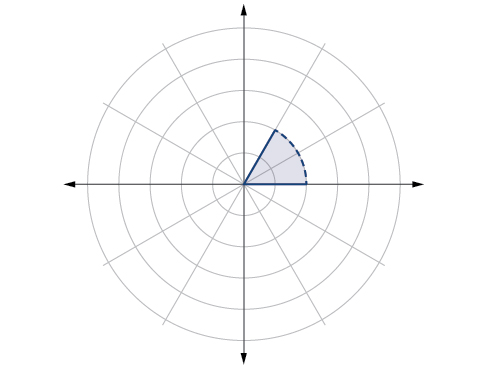$\frac{-\pi }{6}<\theta \le \frac{\pi }{3},-3

A laser rangefinder is locked on a comet approaching Earth. The distance g(x), in kilometers, of the comet after x days, for x in the interval 0 to 30 days, is given by g(x)=250,000csc(π30x). Graph g(x) on the interval [0, 35]. Evaluate g(5)  and interpret the information. What is the minimum distance between the comet and Earth? When does this occur? To which constant in the equation does this correspond? Find and discuss the meaning of any vertical asymptotes.
The sequence is {1,-1,1-1.....} has
how can we solve this problem
Sin(A+B) = sinBcosA+cosBsinA
Prove it
Eseka
Eseka
hi
Joel
June needs 45 gallons of punch. 2 different coolers. Bigger cooler is 5 times as large as smaller cooler. How many gallons in each cooler?
7.5 and 37.5
Nando
find the sum of 28th term of the AP 3+10+17+---------
I think you should say "28 terms" instead of "28th term"
Vedant
the 28th term is 175
Nando
192
Kenneth
if sequence sn is a such that sn>0 for all n and lim sn=0than prove that lim (s1 s2............ sn) ke hole power n =n
write down the polynomial function with root 1/3,2,-3 with solution
if A and B are subspaces of V prove that (A+B)/B=A/(A-B)
write down the value of each of the following in surd form a)cos(-65°) b)sin(-180°)c)tan(225°)d)tan(135°)
Prove that (sinA/1-cosA - 1-cosA/sinA) (cosA/1-sinA - 1-sinA/cosA) = 4
what is the answer to dividing negative index
In a triangle ABC prove that. (b+c)cosA+(c+a)cosB+(a+b)cisC=a+b+c.
give me the waec 2019 questionsBy